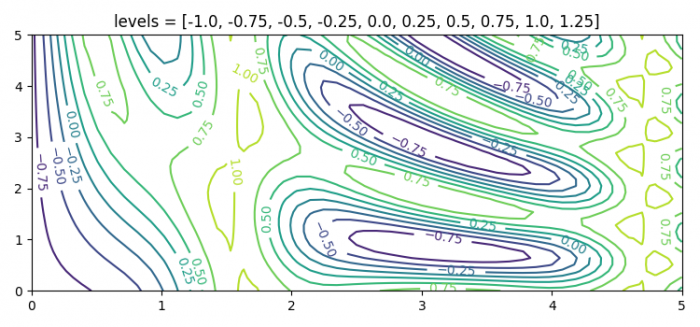# Adding extra contour lines using Matplotlib 2D contour plotting

To add extra contour lines using Matplotlib 2D contour plotting, we can take the following steps −

• Set the figure size and adjust the padding between and around the subplots.

• Create e a function f(x, y) to get the z data points from x and y.

• Create x and y data points using numpy.

• Make a list of levels using Numpy.

• Make a contour plot using contour() method.

• Label the contour plot and set the title of the plot.

• To display the figure, use show() method.

## Example

import matplotlib.pyplot as plt
import numpy as np

plt.rcParams["figure.figsize"] = [7.50, 3.50]
plt.rcParams["figure.autolayout"] = True

def f(x, y):
return np.sin(x) ** 10 + np.cos(10 + y * x) * np.cos(x)

x = np.linspace(0, 5, 50)
y = np.linspace(0, 5, 40)

X, Y = np.meshgrid(x, y)
Z = f(X, Y)
levels = np.arange(-1.0, 1.5, 0.25)
CS = plt.contour(X, Y, Z, levels=levels)

plt.clabel(CS, inline=1, fontsize=10)

plt.title('levels = {}'.format(levels.tolist()))

plt.show()

## Output